# Lambda Calculus

Lambda Calculus adds some extra machinery to expressions to let them take parameters.

Here’s a simple example: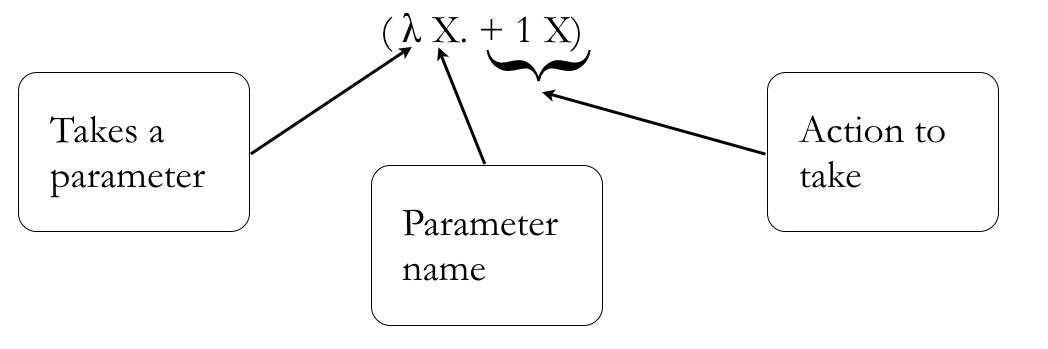Lambda Expression Overview

The λ tells us that this is an expression that has at least one parameter, then we have the name, then a period (.) to indicate that we have got to the end of the list of parameters and, finally, we have the body of the expression, which is the action we should take. What we have here is a function, a reusable piece of code. All λ expressions are functions and the two terms are often used interchangeably. Notice though that these functions don’t have any names – that doesn’t make them very reusable, but that’s something we’ll worry about a bit later on. For now, let’s just use them without names.A lambda expression as an AST. On the right, the same lambda expression with the key parts highlighted.

Notice we still have an AST, noted as the function body on the right hand side, it is just below the λ, which has the parameter name (X) as its other child. Then, inside the body we have the variable name (X). When a value gets passed to the parameter, it will replace the variable, meaning that we will be able to evaluate the AST.

This means we really have two different kinds of expressions. The first is a reducible expression or redex for short. This is an expression that can be made simpler. For example, (+ 2 1) can be reduced to 3. The other type is referred to simply as an expression as λ expression (or function), and can’t be simplified — yet, because it is missing some information. Consider this example (λx. + 1 x).The information we’re missing is what value should be passed in for x; how do we resolve this? We give it a value, like this:

((λx. + 1 x) 5)

Now we have enough information, because we can pass 5 into the parameter, and variable in the body of the expression gets replaced with 5, giving us:

(+ 1 5)

It’s nice and easy from here, as this is just a straightforward prefix expression, and we’re already experts at evaluating (reducing, as we call it now) those.

Let’s run through some more examples:

((λy. * y 5) 2)

(* 2 5)

10

((λxy. + x y) 2 3)

First off, how do we know that there are two variables and not one variable called xy? We know because in λ calculus all variables just have a single letter. To evaluate this, we bring them into the expression in the same order they appear in the list, so x gets the value 2 and gets the value 3. This is called a β (beta) reduction; we’ll come back to that name later on, but it’s just a way of formally describing what we’ve done. In the meantime let’s look at some more examples. How about this?

((λxy. * y x) 2)

Is this a redex? After all, it doesn’t have enough arguments: it needs two but we’ve only given it one. Remember, what makes an expression reducible is the fact that it can be simplified, and we can simplify this performing a β-reduction on it and bringing in the 2 for x to get:

(λy. * y 2)

And that’s as far as we can go, because we now need to wait to get a value for y.

What happens if the argument itself is a redex? Say we had:

((λx. + 3 x) (+ 2 3))

Usually we will evaluate the argument first, so we have this:

((λx. + 3 x) 5)

Next we bring in the 5 and then get our answer of 8, that is, (+ 3 5). However, this won’t always be the case, and sometimes we will bring the argument in unchanged. In this example, the final answer will be the same because we get this:

( + 3 (+ 2 3))

which becomes (+ 3 5). There are some scenarios where this won’t be the case, though, but we’ll worry about those a bit later. For now, let’s draw some ASTs based on the sorts of expressions we’ve been looking at.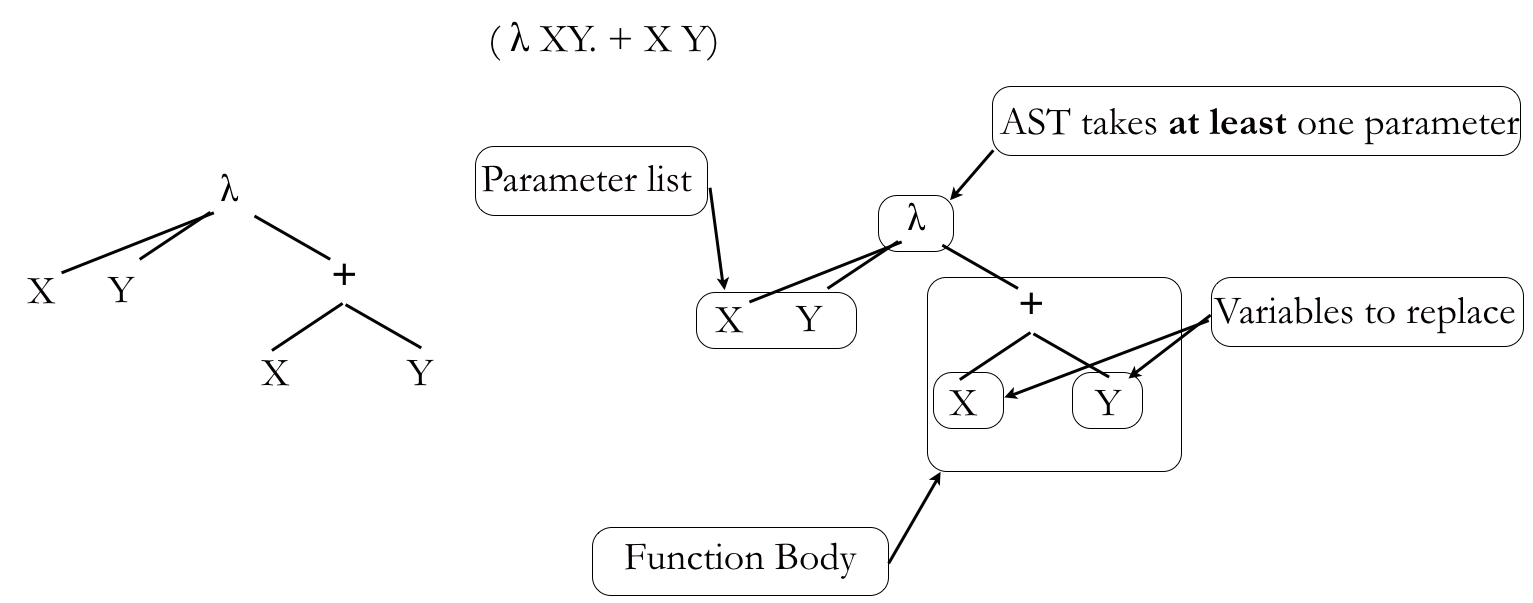A more complex lambda expression as an AST, this time with two parameters. On the right, the same lambda expression with the key parts highlighted.

Now things are starting get a bit more complex, especially once we start passing in other expressions as arguments. We’ll need to know what order to evaluate things in and, for the time being, we will evaluate the innermost redex first, that is, the most deeply nested and furthest to the right.

Consider this one:

((λx. x) (+ 1 1))

The two expressions are at the same depth, but (+ 1 1) is further to the right, so that’s the one we that we will evaluate first. What this means for ASTs is that we need some way to connect these two expressions. After all, we know that separately they look like this: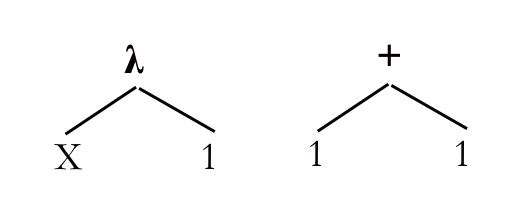Two ASTs, one of which needs to be passed as an argument to the other.

What we need is a way to show that not only are they connected, but how they are connected. In particular, that the (+ 1 1) AST is being passed into (λx. x). In fact, we say that (λx. x) is being applied to (+ 1 1), because (λx. x) is a function. We indicate this with another node, @ (application) that joins the two together, like this:The same ASTs, this time connected with an @ (application) node, showing clearly how they are connected.

Let’s work through some examples. Here we have ((λx. + 1 x) 3):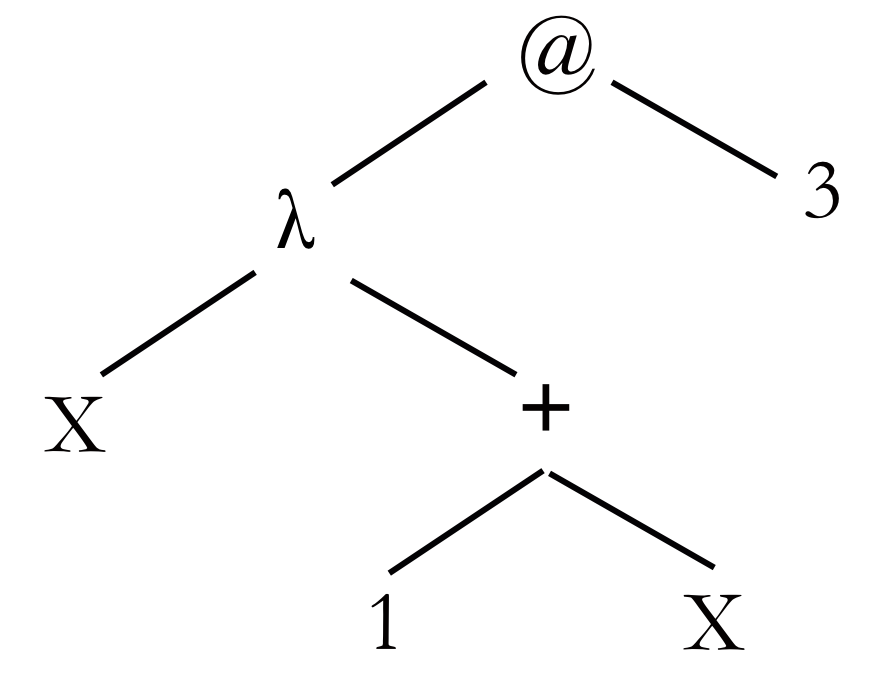An AST with an application node.

The @ on top tells us that we’re going to apply a λ expression to 3, and the  λ expression tells us that we’re going to take one parameter and replace the x in the body with the value we get for it. Let’s bring in that 3 and replace the x: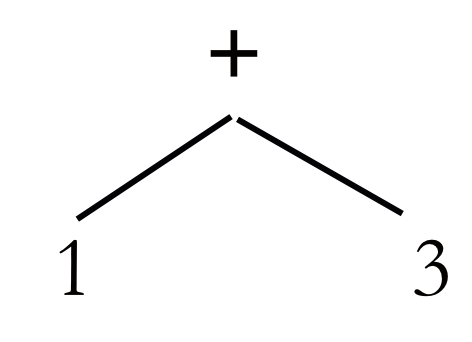The AST after bringing in 3 for X.

This is just a simple AST! We’ve already evaluated far more complex ones that this. This is one of the great things about  λ calculus: if you drill down into it, we have very simple ASTs at the lowest level. Each extra layer, such as the  λ node and the @ node add some extra functionality but they don’t change the fundamentals.

Here’s a slightly more complex example that has two parameters ((λxy. + y x) 1 2):A function with two parameters applied to two arguments

This time we take the arguments into the function in order, that is, in the same order they are presented to the function, so x gets 1 and gets 2, giving us the following AST: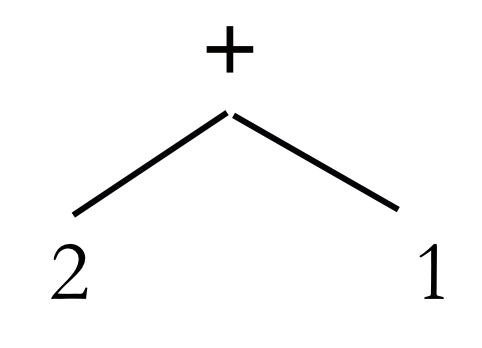The expression with the two values brought in

So, we have three parts to any of these sorts of expressions, we have the function body, that actually does the work, the λ expression, that attaches a parameter list to the function body so that we can supply arguments, and finally, we then apply this to some sort of argument. The following expression ((λx. + 1 x) (+ 4 5)) takes the argument (+ 4 5) and passes it to a function that adds 1 to it: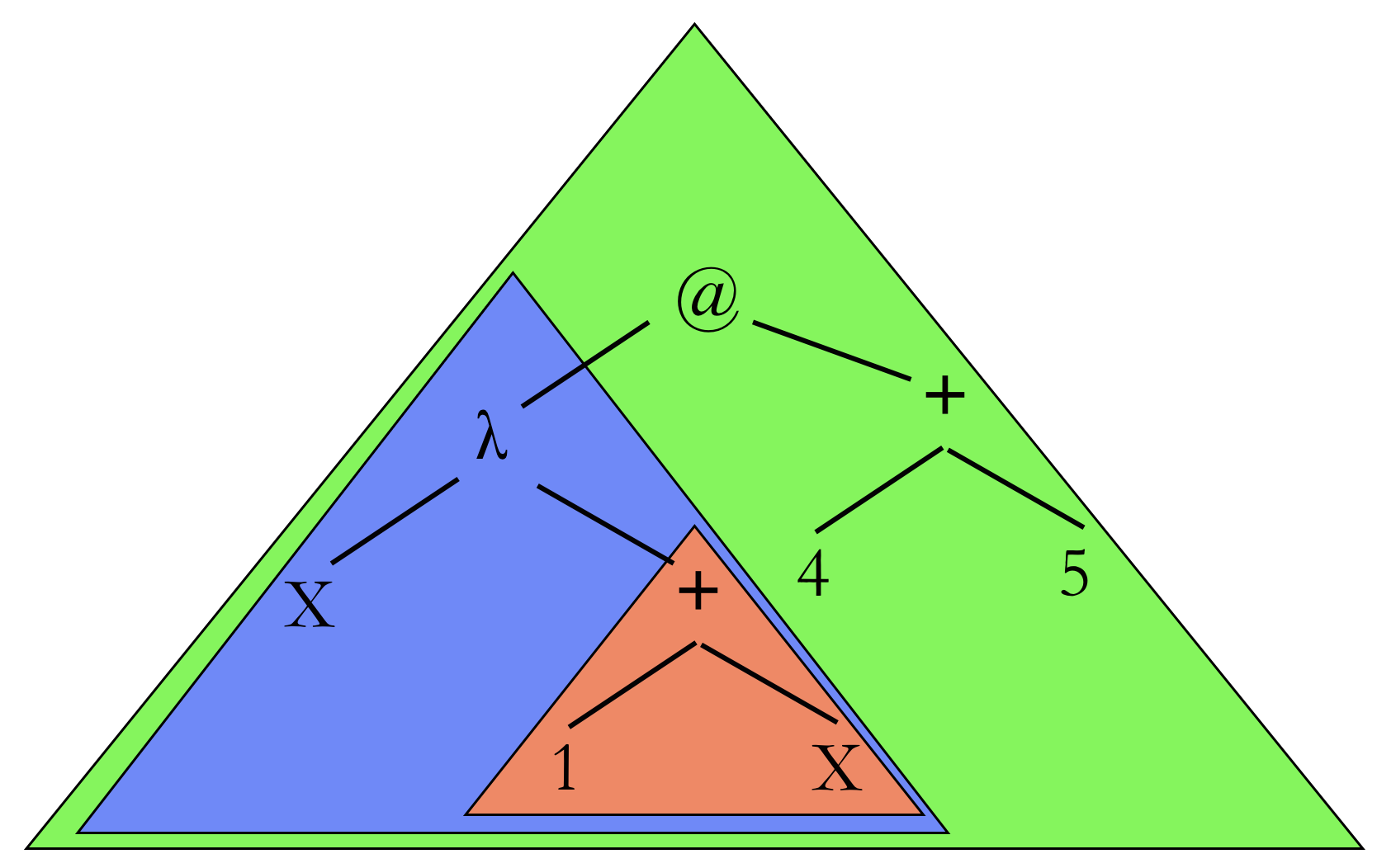The three key parts to an AST with an application node: The body (red triangle); the lambda part (blue triangle) which defines the function; and the application (green triangle) which actually supplies arguments to a function

To evaluate this, we first evaluate the argument — remember, we evaluate the rightmost innermost first:Evaluate the arguments first

Then we replace the x in the function body to give us this: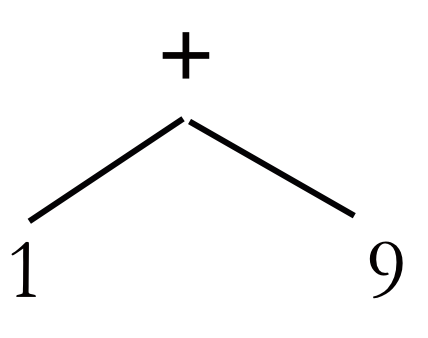The resulting subtree

Take a look at some examples below, which are followed by some practice questions.

Some practice questions on ASTs with λ:

Back to index.